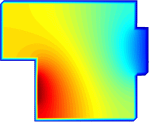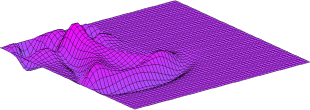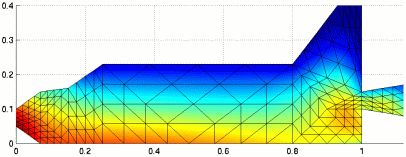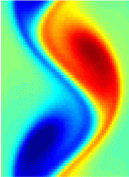McGill University 189-579B Numerical Differential Equations (4 credits) Professor: Martin J. Gander### Course Description

Manyphysical phenomena can be formulated as differential equations, for example the flow of a fluid or the temperature in your room. The solution of these equations is however challenging and analytical methods rarely suffice to obtain the desired results. Only numerical methods can solve the problems of interest in applications.This course introduces you to the theory of modern numerical methods for solving ordinary differential equations (ODEs) and partial differential equations (PDEs). We use throughout the course Matlab to develop prototype codes and we use Maple to avoid tedious hand calculations. This is the ideal course for you if you are an engineer or applied mathematician who needs to solve ODEs and PDEs in your application area. After the course you will be able to make an informed choice when solving your problems numerically.

### Topics

• Numerical methods forordinary differential equations (Linear multi-step and Runge-Kutta methods, consistency, stability and convergence, adaptive integration).
• Hamiltonian problems, symplectic integration.
• Shooting methods for boundary value problems.
• Numerical methods for elliptic partial differential equations, including finite difference, finite element, finite volume and spectral methods.
• The method of lines for hyperbolic and parabolic partial differential equations, implicit and explicit time integration.
• Multi-grid methods and domain decomposition.

###Prerequisites

The main prerequisite for the course is that you understand the continuous problems for which numerical methods are developed. So you need to know ODEs and PDEs at the level taught in a 'methods of mathematical physics' course and basic numerical analysis including programming.

### Time and Location

The course has been scheduled: it is on Monday 18:00-21:00 in Burnside Hall 1205 and starts on January 14th.